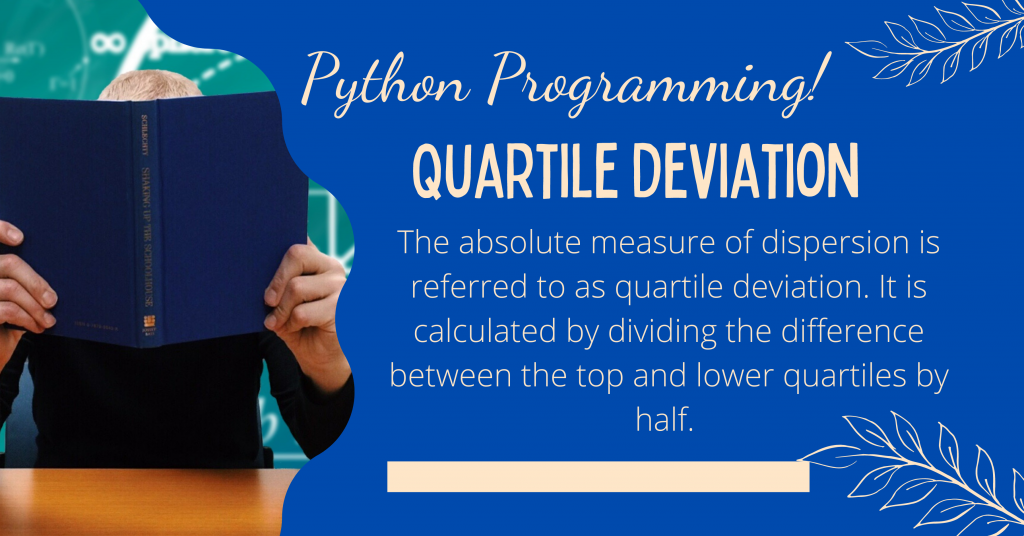# Quartile Deviation – Implementing in PythonHey folks! In this tutorial, I’ll show you how to compute quartile deviation using the Python programming language.

The absolute measure of dispersion is referred to as quartile deviation. It is calculated by dividing the difference between the top and lower quartiles by half.

Also read: Plot Mathematical Functions – How to Plot Math Functions in Python?

## Introduction to Quartile Deviation

The quartile deviation is the absolute measure of dispersion, where dispersion is the amount to which the distribution’s values vary from the mean value.

Even if only one extremely high or low number is present in the data, the range’s utility as a measure of dispersion is diminished.

To compute the quartile deviation, we must divide the data into four sections, each containing 25% of the values.

The quartile deviation of the data is calculated by taking half of the difference between the top (75%) and lowest (25%) quartiles.

## Implementing Quartile Deviation in Python

I hope you now understand what quartile deviation is. Let’s look at how to use Python to determine the quartile deviation of a dataset.

To compute it in Python, we will first build a dataset, then identify the quartile1, quartile2, and quartile3 from the data, and then develop a function that will be useful in returning the product of half the difference between quartile3 and quartile1.

Have a look at the code mentioned below:

```import numpy as np
data = list(range(20, 100, 5))
print("Initial Data : ", data)

Q1 = np.quantile(data, 0.25)
Q2 = np.quantile(data, 0.50)
Q3 = np.quantile(data, 0.75)

print("Quartile 1 : ", Q1)
print("Quartile 2 : ", Q2)
print("Quartile 3 : ", Q3)

def QuartileDeviation(a, b):
return (a - b)/2
print("Computed Result : ",QuartileDeviation(Q3, Q1))
```

## Output of the Code

The code mentioned above will give the following output:

```Initial Data :  [20, 25, 30, 35, 40, 45, 50, 55, 60, 65, 70, 75, 80, 85, 90, 95]
Quartile 1 :  38.75
Quartile 2 :  57.5
Quartile 3 :  76.25
Computed Result :  18.75
```

I hope you enjoyed this tutorial on calculating the quartile deviation of a dataset with the Python programming language.

Read more such tutorials and never stop learning!